AnyLogic

# Dynamic variable

While stock and flow diagrams may contain only stocks and flows, it is a good practice to define intermediate concepts with dynamic variables.

Commonly dynamic variables consist of functions of stocks (and constants or exogenous inputs). In case you need to define a constant, use a parameter.

To create a dynamic variable

1. Drag the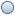Dynamic Variable element from the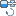System Dynamics palette onto the diagram of agent.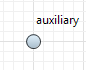2. Go to the Properties view and specify the name of the dynamic variable in the Name edit box.
3. Type the formula for a variable in the variable_name = edit box (you may use code completion here to insert names of other elements).

In case there are some other elements mentioned in the formula, you should draw links from those elements to this dynamic variable first. There is however, link autocreation mechanism supported by AnyLogic — you may just specify the formula — when finished typing, the error indicator will be shown to the left of the formula.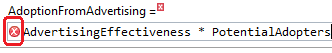Click this indicator to show the pop-up menu with items offering you to create missing links from the mentioned elements to this dynamic variable. Choosing an item from the list you create the corresponding missing link.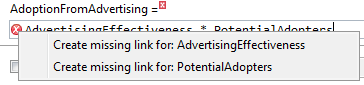You can subsequently create all missing links in such an easy way: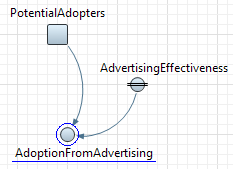4. If you explicitly specify units of measurements for elements of your stock and flow diagram, do it for this dynamic variable. Go to the Advanced section of its properties and select the System dynamics units checkbox and type the unit name in the edit box (you can find more information on units and unit checking in Units.)
5. If you do not want this variable to get its value according constantly re-evaluated formula but otherwise want it to be equal to some specified value (that can be changed during the simulation), check the Constant check box. Constant dynamic variable is drawn with an equal sign over its icon: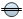.
6. If this dynamic variable has multiple dimensions, declare this dynamic variable as array and then define formulas for its elements as described in the Defining equations for array variables section.
7. If you are creating hierarchical model by exposing system dynamics variables onto interface of the agent type and you want to connect this variable and make it dependent so that it will get its value from the connected variable, select the Dependent check box.
8. Optionally change the fill color of the variable icon by choosing the required color from the Color control.

## Properties

General properties

Name — The name of the dynamic variable. The name is used to identify and access the dynamic variable in formulas.

Show name — If selected, the name of the dynamic variable is displayed on the presentation diagram.

Ignore — If selected, the dynamic variable is excluded from the model.

Visible on upper agent — If selected, the variable is also visible on the upper agent where this agent lives.

Show at runtime — If selected, the variable is visible on the presentation at runtime.

Color — The fill color of the variable icon.

Array — If selected, the variable is of array type. Properties for array variables look slightly different. Instead of one variable_name = field defining the formula for the dynamic variable, there can be several fields defining formulas for some particular elements or sub-arrays of the array variable. To add new section allowing to define one more formula, click the Add formula button. Defining formulas for array elements and sub-arrays is described in the Defining equations for array variables section.

Dependent — If selected, the dynamic variable will get value of the connected variable constantly.

Constant — If selected, the dynamic variable is a constant, i.e. its value does not change according constantly re-evaluated formula but is equal to the specified value (that can be changed by user during the simulation).

variable_name = — The formula defining the value of the dynamic variable. In the case this variable is an array,there can be several fields defining formulas for some particular elements or sub-arrays of the array variable. To add new section allowing to define one more formula, click the Add formula button. Defining formulas for array elements and sub-arrays is described in the Defining equations for array variables section.

Array properties

Array properties are available only if this variable is of array type. To make the variable an array, check the Array check box in the General property section.

Available dimensions — The list of dimensions defined in this model.

Selected dimensions — The list of dimensions that will act as dimensions of this array variable.

These properties are used to define dimensions that make up the array variable. Refer to the Defining an array variable section for details.